## Industry Calculators

• ###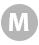M-Weight Calculator

##### Determine the weight of 1,000 sheets of a given sheet size using length, width, and basis weight.
M-WEIGHT = 0 lbs.
(length × width)
length
width
lbs.
(height × width)
height
width

• ###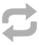MILS/Micron Calculator

##### Convert MILS to Microns and Microns to MILS.
MILS-TO-MICRONS = 0

• ###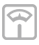Basis Weight Calculator

##### Find the basis weight for a ream of paper based on its weight in grams per square meter.
BASIS-WEIGHT = 0 lbs.
g
(height × width)
length
width

• ###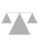Grammage Calculator

##### Find the weight in grams per square meter for a ream of paper based on its basis weight.
GRAMS PER SQUARE METER = 0 g
lbs.
(height × width)
length
width

• ###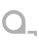Linear Footage Calculator

##### Determine the linear footage of a roll using roll weight and width.
LINEAR FEET = 0
LINEAR YARDS = 0
lbs.
inches
(height × width)
length
width

• ###MSI/MSF Calculator

##### Calculate thousand square inches and thousand square feet of a roll of paper from roll weight and basis weight.
MSI = 0
MSF = 0
lbs.
(height × width)
length
width

• ###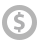Price per MSI/MSF Calculator

##### Derive thousand square-inch price from price per pound and basis weight.
PRICE PER MSI = 0
PRICE PER MSF = 0
(U.S. dollars)
(height × width)
length
width

• ###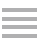Pages-per-Inch Calculator

##### Estimate the number of pages in an inch of paper having a specific thickness, or caliper, per sheet of paper.
PAGES PER INCH = 0
(in decimal inches)

• ###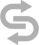Units Conversion Calculator

##### Convert units of measurement typically used in graphic design, such as points to picas, points to milimeters, etc.
RESULT = 0
Convert this quantity

• ###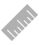Length Conversion Calculator

##### Convert length measurement, such as feet to meters.
RESULT = 0 ?
Convert this quantity

• ###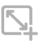Area Conversion Calculator

##### Convert common area measurements, such as acres to square feet.
RESULT = 0 ?
Convert this quantity